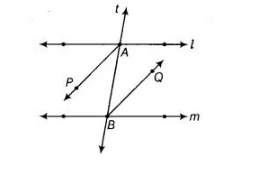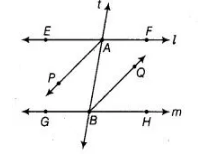# AP and BQ are the bisectors of the two alternate`
Question:

AP and BQ are the bisectors of the two alternate interior angles formed by the intersection of a transversal t with parallel lines l and m (in the given

figure). Show that AP || BQ.Solution:

Given In the figure l || m, AP and BQ are the bisectors of ∠EAB and ∠ABH, respectively.

To prove AP|| BQ

Proof Since, l || m and t is transversal.½ ∠EAB =½ ∠ABH    [dividing both sides by 2]

∠PAB =∠ABQ

[AP and BQ are the bisectors of ∠EAB and ∠ABH] Since, ∠PAB and ∠ABQ are alternate interior angles with two lines AP and BQ and transversal

AB. Hence, AP || BQ.

Therefore, ∠EAB = ∠ABH [alternate interior angles]